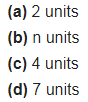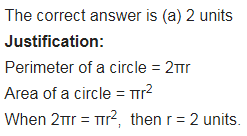# Tick the correct answer in the following and justify your choice:

Tick the correct answer in the following and justify your choice: If the perimeter and the area of a circle are numerically equal, then the radius of the circle is